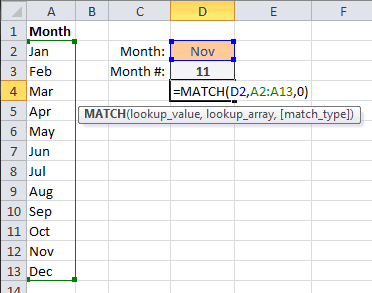The MATCH Function in Excel

The MATCH and INDEX functions working together are more flexible than using the VLOOKUP function. However, if you’ve never used MATCH and INDEX to perform a lookup it can be confusing at first.

I’m going to cover the MATCH function (Excel) here so you can learn it well and don’t have to think about it too much when we put it together with the INDEX function. First comes the dirty details and boring syntax, followed by a couple of examples.

The MATCH Function (Excel) Details

The MATCH function searches for an item in a range of cells and returns its relative position. Or to state another way: the MATCH function returns a position of a matched value from within a range, not the value itself.

The Syntax is:

MATCH(lookup_value, lookup_array, [match_type])

• lookup_value is required and what’s being matched. It can be a number, text, logical value, or reference to one of those three.
• lookup_array is required and should be a one-column or one-row range of cells to be searched.
• match_type is optional. The number -1, 0, or 1.
• If zero (0), MATCH finds and exact match to lookup_value.
• If 1 or omitted, MATCH finds the largest value that is less than or equal to lookup_value, and the lookup_array must be in ascending order.
• If -1, MATCH finds the smallest value that is greater that or equal to lookup_value, and the lookup_array must be in descending order.

The MATCH Function with a Horizontal Lookup Array

The MATCH function in the spreadsheet below looks in cell C3 to get Thu for the lookup_value. The lookup_array is the Day names in the first row, range A1:G1. In the picture, I’m selecting 0 – Exact match for the third argument.The MATCH function returns 5 for Weekday in cell C5 because Thu is found in the fifth column of the range.

The MATCH Function with a Vertical Lookup Array

The MATCH Function in the spreadsheet below, is looking to match Nov, in cell D2, to the Month names in range A2:A13, and finds an exact match in the eleventh row.The MATCH function in cell D3 returns 11, because that’s the position number of Nov in the range of month names.

I could go on, but you get the picture, right?